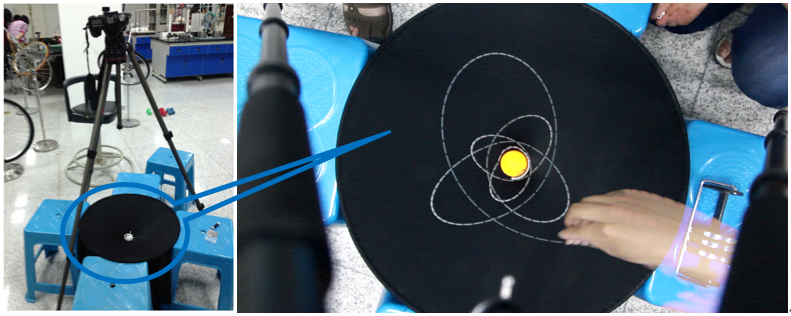# Elastic Space

From the Einstein’s theory of the time and space, our universe will be curved due to the distribution of gravitation. Usually when teaching this concept, the professors will introduce such an experiment: put a heavy ball on the center of the stretched horizontal membrane, and then release some small balls. With the curved surface of the membrane, the small balls will rotate around the center, just like Earth revolves around Sun. The dynamics and apparent interactions of massive balls rolling on a stretched horizontal membrane are often used to illustrate the concept of curved space.

But when we take serious consider of such a system, we will find that it is hard to determine whether this system will be a good indicate for  force like gravitation. Is it possible to define and measure the apparent “gravitational constant” in such a “world”? From this point, we started our research.Figure 1. Overview for the Apparatus and the trace of the small balls

1. Apparatus and Phenomenon

The apparatus of our experiment is simple. We made the membrane tightly scratched, with a heavy ball in the center of the membrane. A high speed camera was arranged for recording. Then we released some small balls with different velocities. Though at first glance, the small balls rotated around the heavy ball, but the trace of the small balls are not enclosed as the conical sections. Thus we could make the first judgment that the ‘gravity force’ in this system is not in the format like F∝1/r^2

2 Theoretical Models:

Since everything in our system follows the Newton’s Law, we can make an analysis based on simple mechanical analysis

We take consider of each circle-ring unit with dr wide. According to the symmetry, the force should towards into the center.Figure 2. Force analysis

For the balance condition, we haveIgnore the O(2) small orderstake T for the membrane to be a constant, Finally we haveThen we take consider of the small balls. If the membrane was smooth, then with the balance condition, we have

3.Experiment Results

First we made a measurement of the membrane shape, with the fitting to its edge, we figure out that the shape is just like the logarithmic function showsFigure 3. the shape and the fitting situation for the membrane

Then we take consider of the traces of small balls. Since the traces are highly depending on the initial situation, so we made several experiment, and made a serial of demonstration via MATLAB. We added the friction analysis in our work. The demonstration and the experiment matched each other perfect:Figure 4. Typical Situations from experiment and the demonstration. The first row comes from the real experiments, and the second row came from the demonstration results of MATLAB.

4.Conclusion and Further Understanding

Our experiment showed the dynamics and apparent interactions of massive balls rolling on a stretched horizontal membrane may not able to illustrate the concept of curved space. But this conclusion can only conduct in the three dimension situation.

Again, if we went back to the analysis of the membrane, we can finally find that the formation of the shape should bewhere k is the elastic constant of the membrane, h(x) is the upward displacement of the membrane (assumed to be small), g is the strength of the gravitational field, and ρ(x) is the mass density of the membrane.

When we further take consider of the gravitation, a Poisson equation will always be introduced:where Φ(x) is the gravitational potential and ρ(x) is the mass density. Again, if we only take the consideration in 2-D world, the formation of the gravitation will beThus, to a first approximation, a massive object placed on a membrane sheet will deform the sheet into a correctly shaped gravity well, but this is just like the demonstration for a 2-D world. In other word, the curved space in 3-D world will generate the same situation of gravity in 2-D world. Similarly, we can imagine the curved space in 4-D world will generate the gravitation in our 3-D world, just like Einstein assumed.